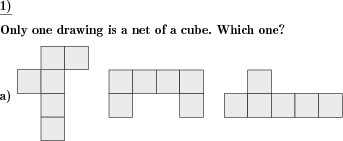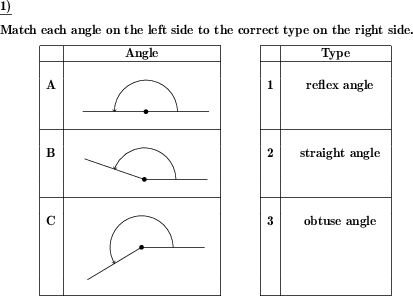Custom math worksheets at your fingertipsDetails for problem "Identify cube nets in a series of cube-like nets"

Quickname: 1011

Elementary School, Primary School, Middle School.

Summary

A series of drawings is shown. Which ones represent cube nets?

ExampleDescription

A series of shapes, of nets, is shown. Some of the nets are cube nets, the others are not. The cube nets have to be identified.

The number of problems, that is the number of series of cube nets, can be selected. The number of presented nets in each problem can be chosen, as can be the number of actual cube nets.

For a lower level of difficulty, the actual number of nets in the series of shapes can be indicated to the reader.

Download free printable worksheets for this math problem here. The worksheet contains the problems only, the solution sheet includes the answers. Just click on the respective link.

•Worksheet 1Solution sheet with answers
•Worksheet 2Solution sheet with answers
•Worksheet 3Solution sheet with answers

If you can not see the solution sheets for download, they may be filtered out by an ad blocker that you may have installed. If this is the case, please allow ads for this page and reload the page. The solution sheets will then reappear.

• Do these sample worksheets do not really fit?
• Do you need more math worksheets, with a different level of difficulty?
• Would you like to combine different problems on a worksheet and adjust them to your needs?
• As a teacher, you can put together your own worksheets using the automatically generated math problems provided.
With a free initial credit, you can start creating your own math worksheets in a few minutes.

You can try it for free! Register here, to create custom worksheets now!

Customization options for this problem

Parameter
Possible values
Number of problems
1, 2, 3
Number of nets
1, 2, 3, 4, 5, 6
Cube nets thereof
1, 2, 3, 4
State number of nets
Yes, No

Other types of problems that appear on worksheets with this problem:

Relevance
Name
Description
Quickname
Example
****
Angle types
For a given angle, the correct type has to be marked.****
Match angles to angle types
Given angles have to be matched to given types****
Triangle label parts
A fully or partly unlabeled triangle is given. The vertices, sides or angles have to be labeled correctly.****
Triangle draw incircle
The incircle and angle bisectors have to be drawn for a given triangle.Deutsche Version dieser Aufgabe
These informational pages with samples describe math problems that can be combined on custom math worksheets with solutions for home and K-12 school use.
Deutsche Seiten
×